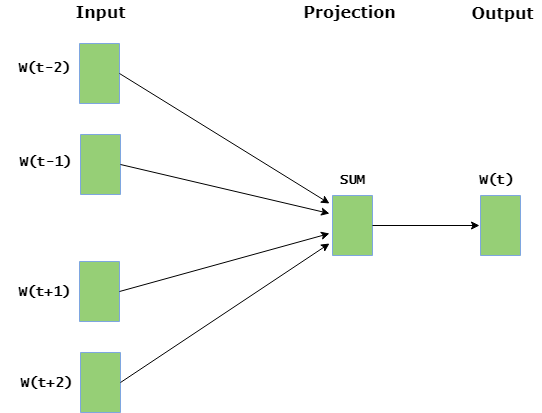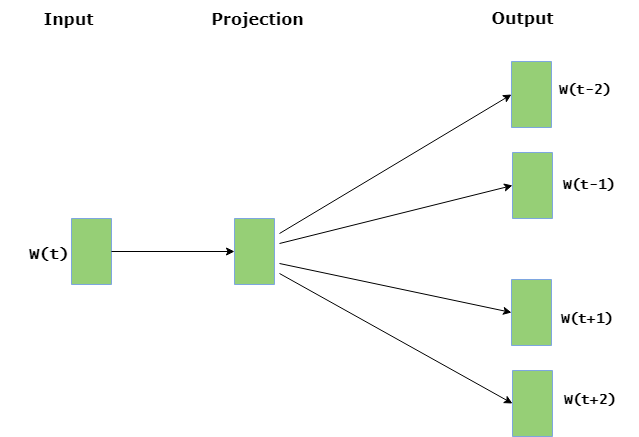# Python | Word Embedding using Word2Vec

Word Embedding is a language modeling technique used for mapping words to vectors of real numbers. It represents words or phrases in vector space with several dimensions. Word embeddings can be generated using various methods like neural networks, co-occurrence matrix, probabilistic models, etc.

Word2Vec consists of models for generating word embedding. These models are shallow two layer neural networks having one input layer, one hidden layer and one output layer. Word2Vec utilizes two architectures :

1. CBOW (Continuous Bag of Words) : CBOW model predicts the current word given context words within specific window. The input layer contains the context words and the output layer contains the current word. The hidden layer contains the number of dimensions in which we want to represent current word present at the output layer.2.

3. Skip Gram : Skip gram predicts the surrounding context words within specific window given current word. The input layer contains the current word and the output layer contains the context words. The hidden layer contains the number of dimensions in which we want to represent current word present at the input layer.The basic idea of word embedding is words that occur in similar context tend to be closer to each other in vector space. For generating word vectors in Python, modules needed are `nltk `and `gensim`.

Run these commands in terminal to install `nltk ` and `gensim` :

```pip install nltk
pip install gensim```

Download the text file used for generating word vectors from here .

Below is the implementation :

 `# Python program to generate word vectors using Word2Vec ` ` `  `# importing all necessary modules ` `from` `nltk.tokenize ``import` `sent_tokenize, word_tokenize ` `import` `warnings ` ` `  `warnings.filterwarnings(action ``=` `'ignore'``) ` ` `  `import` `gensim ` `from` `gensim.models ``import` `Word2Vec ` ` `  `#  Reads ‘alice.txt’ file ` `sample ``=` `open``(``"C:\\Users\\Admin\\Desktop\\alice.txt"``, ``"r"``) ` `s ``=` `sample.read() ` ` `  `# Replaces escape character with space ` `f ``=` `s.replace(``"\n"``, ``" "``) ` ` `  `data ``=` `[] ` ` `  `# iterate through each sentence in the file ` `for` `i ``in` `sent_tokenize(f): ` `    ``temp ``=` `[] ` `     `  `    ``# tokenize the sentence into words ` `    ``for` `j ``in` `word_tokenize(i): ` `        ``temp.append(j.lower()) ` ` `  `    ``data.append(temp) ` ` `  `# Create CBOW model ` `model1 ``=` `gensim.models.Word2Vec(data, min_count ``=` `1``,  ` `                              ``size ``=` `100``, window ``=` `5``) ` ` `  `# Print results ` `print``(``"Cosine similarity between 'alice' "` `+`  `               ``"and 'wonderland' - CBOW : "``, ` `    ``model1.similarity(``'alice'``, ``'wonderland'``)) ` `     `  `print``(``"Cosine similarity between 'alice' "` `+` `                 ``"and 'machines' - CBOW : "``, ` `      ``model1.similarity(``'alice'``, ``'machines'``)) ` ` `  `# Create Skip Gram model ` `model2 ``=` `gensim.models.Word2Vec(data, min_count ``=` `1``, size ``=` `100``, ` `                                             ``window ``=` `5``, sg ``=` `1``) ` ` `  `# Print results ` `print``(``"Cosine similarity between 'alice' "` `+` `          ``"and 'wonderland' - Skip Gram : "``, ` `    ``model2.similarity(``'alice'``, ``'wonderland'``)) ` `     `  `print``(``"Cosine similarity between 'alice' "` `+` `            ``"and 'machines' - Skip Gram : "``, ` `      ``model2.similarity(``'alice'``, ``'machines'``)) `

Output :

```Cosine similarity between 'alice' and 'wonderland' - CBOW :  0.999249298413
Cosine similarity between 'alice' and 'machines' - CBOW :  0.974911910445
Cosine similarity between 'alice' and 'wonderland' - Skip Gram :  0.885471373104
Cosine similarity between 'alice' and 'machines' - Skip Gram :  0.856892599521
```

Output indicates the cosine similarities between word vectors ‘alice’, ‘wonderland’ and ‘machines’ for different models. One interesting task might be to change the parameter values of ‘size’ and ‘window’ to observe the variations in the cosine similarities.

```Applications of Word Embedding :

>> Sentiment Analysis
>> Speech Recognition
>> Information Retrieval
```

References :

My Personal Notes arrow_drop_upCheck out this Author's contributed articles.

If you like GeeksforGeeks and would like to contribute, you can also write an article using contribute.geeksforgeeks.org or mail your article to contribute@geeksforgeeks.org. See your article appearing on the GeeksforGeeks main page and help other Geeks.

Please Improve this article if you find anything incorrect by clicking on the "Improve Article" button below.

Article Tags :

2

Please write to us at contribute@geeksforgeeks.org to report any issue with the above content.